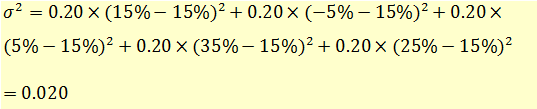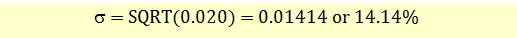# Calculating Variance and Standard Deviation of Stock Returns

We can also calculate the variance and standard deviation of the stock returns. The variance will be calculated as the weighted sum of the square of differences between each outcome and the expected returns.The standard deviation will be:Remember that the units of measuring standard deviation are the same as the units of measuring stock returns, in this case percentage (%).